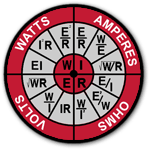# Calculators

All of our electric heater calculators in one place.

Power Flowrate Temp Calculator

Calculate the electrical power, flow rate or temperature requirement as follows:

• SCFM = airflow in standard cubic feet per minute
• ΔT = temperature rise in degrees F from the inlet to the exhaust
• Watts = SCFM x ΔT/3

Fill in two values to determine the third

Temperature Conversion Calculator

°F = ( ( ( °C * 9) / 5 ) + 32 )
°C = ( ( ( °F - 32) * 5 ) / 9 )

Fill in one value to calculate the other

Three Phase Unit Calculator

Line Current = Wattage / (Voltage * √3)

Fill in two values to determine the third

Single Phase Unit Calculator

Line Current = Wattage / Voltage

Fill in two values to determine the third

Ohms Law CalculatorFill in two values to determine the other two

Heat Transfer Through Convection Calculator
• ρ = density (lb/ft3)
• V = volume flow rate (ft3/hour)
• Cp = specific heat (Btu/lb°F)
• Ta-Tb = temperature differential (°F)
• Q = ρ x V x Cp x (Ta-Tb)

Fill in four values to determine the fifth

ACFM to SCFM Calculator
• ACFM = airflow in actual cubic feet per minute
• P = gage pressure (psi)
• T = gas temperature °R = 460 + °F
• SCFM = airflow in standard cubic feet per minute

Fill in 3 values to determine the SCFM

Mass Flow to Volumetric Flow Calculator

Mass flow rate to Volumetric flow rate for air

Fill in one value to calculate the other two

## Enclosure Heater Calculator

### Is another enclosure adjacent to this enclosure?Calculators
Calculators +